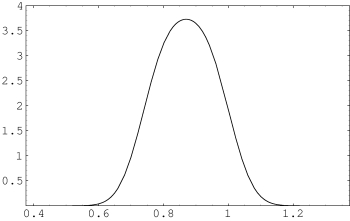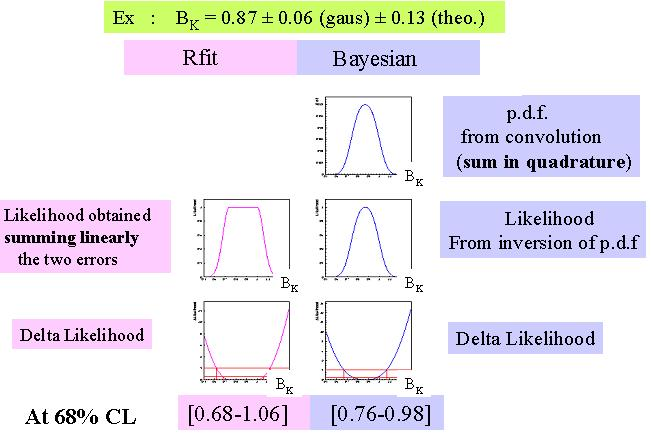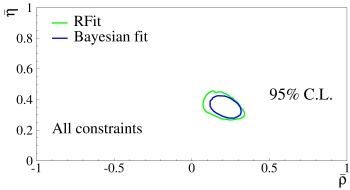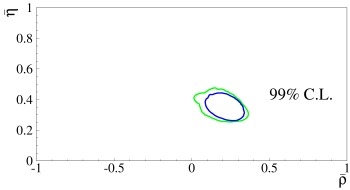# Description of the Bayesian method

The method is explained in detail in M. Ciuchini et al., JHEP 0107 (2001) 013, hep-ph/0012308.

Given N parametersand M constraints, whose actual value depends onand on CKM parameters, what is the best determination of? Bayes Theorem tells us that:whereis the p.d.f. for the constraints or parameters andis the a-priori probability for.

The output p.d.f. foris obtained by integrating over the parameters space:Several remarks are in order:
• The present knowledge of each quantity is expressed with a p.d.f. or, if you prefer, with a Likelihood function (in the Bayesian approach is always possible to define a p.d.f. as).
• The method does not make any distinction (formally) between theoretical and experimental parameters or between Gaussian and non-Gaussian distributions. It can easily digest any kind of p.d.f./Likelihood (unlike other methods).
• The Bayesian concept of updating the knowledge is naturally applied. Starting from no information on the actual value ofand, one can update (and improve) it using the available experimental and theoretical information.
How do we treat inputs?
• When available, we use the experimental likelihoods.
• If a parameter has an uncertainty given as "allowed range" we assume this parameter to be uniformly distributed in the range. This could apply in principle both to theoretical estimates or to experimental systematics.
The final p.d.f. of the parameter is computed as a convolution between all the uncertainties. Consider the following example of a p.d.f. for a theoretical parameter:• The most relevant (but unavoidable) assumption is on the size of the range (flat part) of the theoretical uncertainty, not in the shape of the p.d.f.
• The central value is preferred (as expected).
The interpretation of the result of a Bayesian fit is well defined probabilistically, since the output of the fit is given in terms of p.d.f.'s.
• The allowed regions are well defined in term of probability. Allowed regions at 95% mean that you expect the "true" value in this range with a 95% probability (and not "at least 95%" as, by definition, in the frequentist approaches).
• Any p.d.f. can be extracted by changing the integration variables → indirect determination of any interesting quantity (theoretical parameter, unmeasured quantities)

# Other methods

Other statistical approaches are based on a frequentist understanding of systematic theoretical uncertainties, which cannot be treated as statistically distributed quantities. In this framework two main approaches can be distinguished : the Rfit method and the Scanning method. In both methods, the "theoretically allowed" values for some theoretical parameters are "scanned", i.e. no statistical weight is assigned to these parameters as long as their values are inside a "theoretically allowed" range.
At present three groups are producing results in this framework:
The Rfit and the Scan methods are also described in Chapter V of the Yellow Report (CERN-EP/2003-002, hep-ph/0304132).

# Comparison between Rfit and Bayesian methods

The comparison between Rfit and Bayesian methods has been done during the first CKM Workshop held at CERN on 13-16 February 2002. The results are reported in Chapter V of the Yellow Report (CERN-EP/2003-002) ( hep-ph/0304132). We summarize here the main results.
The aim of this comparison was to evaluate the difference in the output quantities as obtained from the two statistical methods and not to judge their validity (statistical foundation). At the Workshop it was concluded that the output results differ mainly because of the different treatment of the input quantities.

## Treatment of the input quantities

The treatment of the inputs differ between the two methods. The uncertainty on a quantity is usually split in two parts: a statistical part which can be described by a Gaussian p.d.f., (this part may contain many sources of uncertainties which have been already combined into a single p.d.f.) and another part which is usually of theoretical origin and is often related to uncertainties due to theoretical parameters. In the following we will denote it as theoretical systematics. It is often described using an uniform p.d.f. The resultant p.d.f. is obtained from the convolution of the two p.d.f.'s, which is, obviously, not necessarily a Gaussian p.d.f.
In the frequentist analysis, no statistical meaning is attributed to the uncertainties related to theoretical systematics. The likelihood function which describes this quantity is obtained as a product between this uncertainty and the statistical one (which corresponds to a linear sum of the errors).
In conclusion even if the inputs used are the same in the two approaches (in term of Gaussian and uniform uncertainties), they correspond to different input likelihoods. An example, using the quantity BK, is given below.Table of inputs used for this comparison
 Constraints, Parameters Value Gaussian Error Flat Error Comments0.762 0.064 - -0.2210 0.0020 - -42.1 2.1 - Average of exclusive40.4 0.7 0.8 Average of inclusive32.5 2.9 5.5 For the moment → only CLEO40.9 4.6 3.6 For the moment → LEP + CLEO endpoint0.494 0.007 - WA (CDF/CLEO/LEP/BaBar/Belle)>14.5 @ 95% C.L. - - Sensitivity at 19.3 (CDF/LEP/SLD)The Likelihood Ratio is used.167 5 - (CDF/D0)230 30 15 Lattice QCD1.18 0.03 0.04 Lattice QCD

## Comparison of output quantities

For the comparison of the results of the fit we use,,and. Those quantities are compared at the 95%, 99% and 99.9% C.L.. It has to be stressed that in the frequentist approach those confidence levels correspond to >95%, >99% and >99.9%.Allowed regions for. The closed contours at 95% C.L. and 99% C.L. on the left and right plots respectively. The green and the blue contours are obtained using the Rfit and Bayesian methods, respectively. The constraints used are:,,,, and. The ratio Rfit/Bayesian between the ranges, at different C.L., for the most relevant output quantities, is given in the Table below.
 Parameter 95% C.L. 99% C.L. 99.9% C.L.1.43 1.34 1.121.18 1.12 1.051.17 1.18 1.161.46 1.31 1.09

The origin of the residual difference between the two methods has been further tested performing the following tests: both methods use the distributions as obtained from Rfit or from the Bayesian method to account for the information on input quantities. The results of the comparison using the input distributions as obtained from Rfit are shown in the Figures below and the results are summarized in the Table below. In some cases (99.9% and 99% C.L.) the ranges selected by the Bayesian approach are wider. The comparison using the input distributions, as obtained from the Bayesian method, give a maximal difference of 5%.Parameter 95% C.L. 99% C.L. 99.9% C.L.1.20 1.13 0.961.03 0.99 0.941.07 1.08 1.071.24 1.12 0.95

These two tests show that, if same input likelihoods are used, the results on the output quantities are very similar. The main origin of the residual difference on the output quantities, between the Bayesian and the Rfit method comes from the likelihood associated to the input quantities.

We report here the conclusion as written in Chapter V of the Yellow Report (CERN-EP/2003-002) (hep-ph/0304132).

"The Bayesian and the Rfit methods are compared in an agreed framework in terms of input and output quantities. For the input quantities the total error has been split in two errors. The splitting and the p.d.f distribution associated to any of the errors is not really important in the Bayesian approach. It becomes central in the Rfit approach where the systematic errors are treated as "non statistical" errors. The result is that, even if the same central values and errors are used in the two methods, the likelihood associated to the input parameters, which are entering in the fitting procedure, can be different. The output resultsdiffer by 15%-45%, 10%-35% and 5-15% if the 95%, 99% and 99.9% confidence regions are compared, respectively, with ranges from the frequentist method being wider. If the same likelihoods are used the output results are very similar.

Ideas, requests, problems regarding this web site? Send feedback